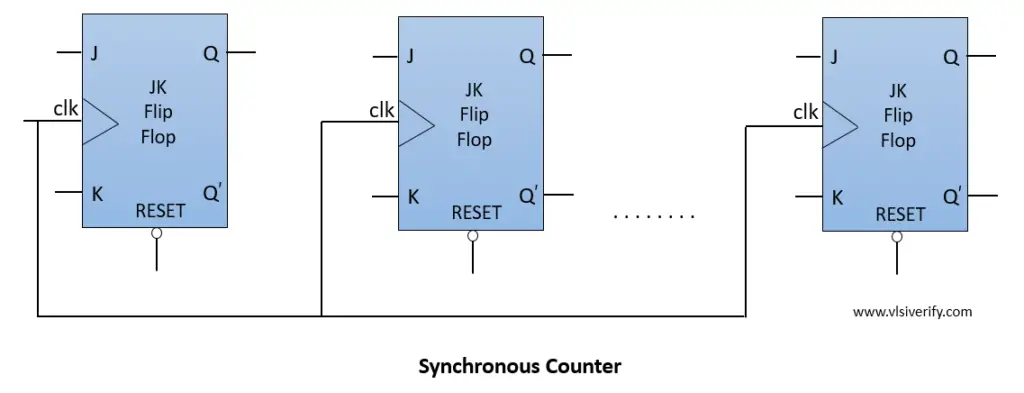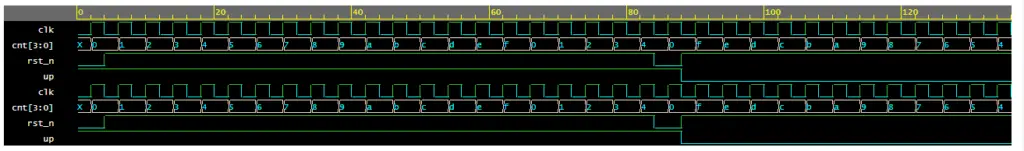Tutorials

As we have discussed the asynchronous counter, output of one flip-flop stage is connected to the input clock pin of the next stage that introduces propagation delay. In a synchronous counter, all flip-flops within the counter are clocked together by the same clock which makes it faster when compared with an asynchronous counter. It is known as a parallel counter.

## Block Diagram## 4-bit Up-Down Synchronous Counter

``````module synchronous_counter #(parameter SIZE=4)(
input clk, rst_n,
input up,
output reg [3:0] cnt
);

always@(posedge clk) begin
if(!rst_n) begin
cnt <= 4'h0;
end
else begin
if(up) cnt <= cnt + 1'b1;
else cnt <= cnt - 1'b1;
end
end
endmodule``````

## Testbench Code

``````module tb;
reg clk, rst_n;
reg up;
wire [3:0] cnt;
synchronous_counter(clk, rst_n, up, cnt);

initial begin
clk = 0; rst_n = 0;
up = 1;
#4; rst_n = 1;
#80;
rst_n = 0;
#4; rst_n = 1; up = 0;
#50;
\$finish;
end
always #2 clk = ~clk;

initial begin
\$dumpfile("dump.vcd"); \$dumpvars;
end
endmodule``````

## Waveform## Difference between synchronous and asynchronous counter

 Asynchronous Counter Synchronous Counter In asynchronous counter, the output of one flip flop stage is driven to the clock input of the next stage. In the synchronous counter, the same clock is driven to all flip-flop stages Slower as compared to the synchronous counter Faster as compared to the asynchronous counter. It is also known as serial counter It is also known as parallel counter High propagation delay Propagation delay is less compared to the asynchronous counter.

Verilog Codes